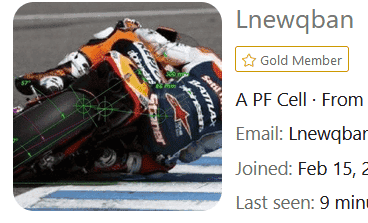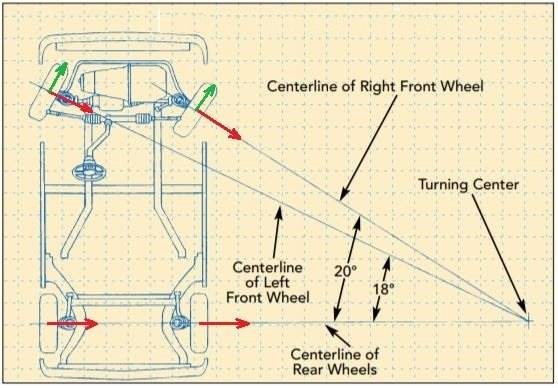# Direction of friction of each wheel and total moment when a car turns

• I
• JM7

#### JM7

Static friction is known to provide centripetal force when a car turns.
Assuming uniform circular motion, my questions are
1. Is the static friction of each wheel points toward the center of turning circle or it's the combined forces of all four wheels that has to point toward the center of turning circle from the center of mass of the car?
2. Would the total moment of frictional force of all four wheels with respect to the center of mass be zero (no net torque so no angular acceleration)?
3. Is there any condition for the relative values between each frictional force e.g. the frictional forces of the two rear wheels have to be the same or the frictional forces of the inner (front/rear) wheel are always higher than those of the outer (front/rear) wheel?

Welcome!1. The static friction of each wheel points toward the center of the turning circle.

2. Ideally, the total moment of frictional force of all four wheels with respect to the center of mass is zero. In reality, some cars are over-turning, and some are under-turning, according to the location of the center of mass, and how the tires deform under lateral loads.

3. The frictional forces of the inner (front/rear) wheel are always lower than those of the outer (front/rear) wheels, since there is a rolling torque or moment (the center of mass is higher than the contact patches).

https://en.wikipedia.org/wiki/Vehicle_dynamics

https://en.wikipedia.org/wiki/Automobile_handling

https://en.wikipedia.org/wiki/Body_roll

https://en.wikipedia.org/wiki/Weight_transfer

https://en.wikipedia.org/wiki/Understeer_and_oversteerLast edited:
1. Why is the direction of static friction of each wheel not tangential to the direction of the wheel? Consider a front-wheel drive car which is moving along a straight path, the direction of the static friction of the rear wheels are opposite the motion of the wheels (and the car). Would the static friction of the rear wheels change direction quite suddenly by 90 degrees when the car starts to turn?
2. Shouldn't the static friction of all four wheels be added up to be the centripetal force mv^2/R pointing towards the center from the center of the mass?

1. Why is the direction of static friction of each wheel not tangential to the direction of the wheel? Consider a front-wheel drive car which is moving along a straight path, the direction of the static friction of the rear wheels are opposite the motion of the wheels (and the car). Would the static friction of the rear wheels change direction quite suddenly by 90 degrees when the car starts to turn?
2. Shouldn't the static friction of all four wheels be added up to be the centripetal force mv^2/R pointing towards the center from the center of the mass?
1. The direction of static friction of each wheel is aligned with the direction of the wheel during acceleration (driving wheels) and braking (driving and driven wheels) on a straight line.
For a non-braking driven wheel, there is the rolling friction, which makes it freely roll over the pavement.
The static friction of any wheel becomes a combination (or vectorial addition) of any tangential or longitudinal friction force (mentioned above) and a lateral friction force after the car starts to turn.

2. The lateral static friction of all four wheels should be added up to equal the centripetal force mv^2/R pointing towards the center from the center of the mass.
https://en.wikipedia.org/wiki/Circle_of_forces

http://www.formula1-dictionary.net/traction_circle.html

https://en.wikipedia.org/wiki/Cornering_force

You are welcome1. Is the static friction of each wheel points toward the center of turning circle or it's the combined forces of all four wheels that has to point toward the center of turning circle from the center of mass of the car?
2. Would the total moment of frictional force of all four wheels with respect to the center of mass be zero (no net torque so no angular acceleration)?
3. Is there any condition for the relative values between each frictional force e.g. the frictional forces of the two rear wheels have to be the same or the frictional forces of the inner (front/rear) wheel are always higher than those of the outer (front/rear) wheel?
Welcome to PF.

@Lnewqban is obiously simplifying his replies for your question. As you can see from his avatar, his force vector involves a very signifcant tantential acceleration as well as the radial centripital acceleration...•Lnewqban
1. But if the static friction of each wheel points toward the center (from your earlier answer), doesn't that mean that the tangential component of the static friction (of each wheel) becomes zero?
2. So are the following conditions required at the same time
(a) the static friction of each wheel points toward the center
(b) the vectorial sum of the static friction of all four wheels points toward the center
Thank you.

Last edited:
1. But if the static friction of each wheel points toward the center (from your earlier answer), doesn't that mean that the tangential component of the static friction (of each wheel) becomes zero?
2. So are the following conditions required at the same time
(a) the static friction of each wheel points toward the center
(b) the vectorial sum of the static friction of all four wheels points toward the center
Thank you.